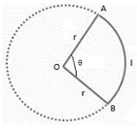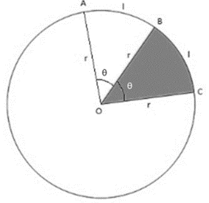# Area of a Sector of a Circle Formula

## Area of a Sector of a Circle Formula - Solved Questions & Examples

A sector of a circle is a closed figure bounded by an arc of a circle and two of its radii. Each sector has a unique central (sector) angle that it subtends at the center of the circle. Minor sectors subtend angles less than 180° while major sectors subtend angles more than 180°. The semicircular sector subtends an angle of 180°. The following diagram shows a minor sector of a circle of radius r units whose central angle is θ.Firstly, it is important to realize that a circle is a perfectly symmetric planar figure. It has infinite lines of symmetry passing through its centre. This means that all sectors, of the same circle or of congruent circles, which have the same central angles are congruent sectors. We will use this fact to derive the formulas for the perimeter and the area of a sector. Consider the following diagram which shows two adjacent congruent sectors of a circle.Sectors OAB and OCB have the same central angle (θ), hence their arc lengths AB and BC are equal (l), as well as their areas. Together they form a bigger sector OAC. Clearly the central angle of sector OAC is , while its arc length AC is 2l . Therefore, we realize that the arc length of a sector is directly proportional to its central angle. The same applies for the area of the sector as well.
So let’s consider a sector of a circle of radius r and central angle θ. We know that for a central angle of 360°, the sector is actually the complete circle. So we can say that for a central angle of 360°, the sector’s arc length is equal to the perimeter of the circle and its area is equal to the area of the circle. Thus, using the concept of direct proportions, we arrive at the following results.
$\frac{l}{\theta } = \frac{{2\pi r}}{{{{360}^ \circ }}} \Rightarrow l = \frac{\theta }{{{{360}^ \circ }}} \times 2\pi r$
$\frac{{area}}{\theta } = \frac{{\pi {r^2}}}{{{{360}^ \circ }}} \Rightarrow area = \frac{\theta }{{{{360}^ \circ }}} \times \pi {r^2}$
The term $\frac{\theta }{{{{360}^ \circ }}}$ can be thought of as the fraction of the total central angle of the circle (360°) covered by the sector. We can even relate the area of the sector to its arc length by using the above two formulas to obtain a simple formula for the area, as shown below.
$area = \frac{\theta }{{{{360}^ \circ }}} \times \pi {r^2} = \frac{1}{2} \times \frac{\theta }{{{{360}^ \circ }}} \times 2\pi r \times r = \frac{1}{2}lr$
To find the perimeter of the sector, we add the lengths of the two radii to the arc length.
${\text{perimeter}} = l + 2r$
Let’s look at an example to see how to use these formulas.

Question: Find the perimeter and the area of a sector of a circle of radius 35 cm whose central angle is 72°.
Solution:
$r = 35\,cm,\,\,\theta = {72^ \circ }$
$l = \frac{\theta }{{{{360}^ \circ }}} \times 2\pi r = \frac{{72}}{{360}} \times 2 \times \frac{{22}}{7} \times 35 = 44\,cm$
$perimeter = 2r + l = 2 \times 35 + 44 = 114\,cm$
$area = \frac{1}{2}lr = \frac{1}{2} \times 44 \times 35 = 770\,c{m^2}$
Why don’t you try solving the following problem to see if you have mastered using these formulas?

Question: Find the central angle of a sector of a circle whose area and perimeter are respectively equal to $231\,c{m^2}$ and $61\,cm$ if it is given that its radius is a whole number.
Options:
(a) $10{5^o}$
(b) $12{0^o}$
(c) $13{5^o}$
(d) none of these
${\text{area}} = 231\,c{m^2},\,\,\,\,{\text{perimeter}} = 61\,cm$
${\text{perimeter}} = 2r + l = 61$
${\text{area}} = \frac{1}{2}lr = 231 \Rightarrow lr = 462$
$\begin{gathered} \left( {61 - 2r} \right) \times r = 462 \hfill \\ \Rightarrow 61r - 2{r^2} = 462 \hfill \\ \Rightarrow 2{r^2} - 61r + 462 = 0 \hfill \\ \Rightarrow 2{r^2} - 28r - 35r + 462 = 0 \hfill \\ \Rightarrow \left( {2r - 33} \right)\left( {r - 14} \right) = 0 \hfill \\ \Rightarrow r = 16.5\,cm,\,\,14\,cm \hfill \\ \end{gathered}$
Since r is given to be a whole number, $r = 14\,cm$
$\begin{gathered} \frac{\theta }{{{{360}^ \circ }}} \times \frac{{22}}{7} \times {14^2} = 231 \hfill \\ \Rightarrow \theta = {360^ \circ } \times 7 \times \frac{{231}}{2} \times 14 \times 14 = {135^ \circ } \hfill \\ \end{gathered}$